# Money + area - math problems

#### Number of problems found: 36

• Matilda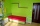Matilda measures the perimeter of her bedroom to determine how many feet of border she needs to go around the tops of the walls. Two walls are each 96 inches long, and two walls are each 3 yards long. She pays \$30 for border that costs \$1 per foot. What a
• Mrs Holland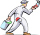Mrs Holland wants to paint her garage wall. The wall measures 6 m x 3 m Each can of paint covers 5m². Each can costs £7.50 How much will it cost Mrs Holland to paint her garage wall?
• Living roomMarina's new living room is a rectangle with a length of 8 meters and a width of 5 meters. She wants to buy a carpet to cover half of the living room and each square meter of the carpet has a cost of \$4. How much will be the total cost for Marina?
• The kitchenThe kitchen has the shape of a square with a side of 5 m. We place linoleum on the floor, the price of which is 450 CZK per 1 m2. The glue costs 750 CZK. How much do we pay in total?
• HectareThe rectangular plot has a width of 2500 cm and is 0.065 km long. How many CZK will we pay for covering the land with a lawn at the price of CZK 3,500 per hectare?
• Carpet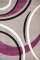How many crowns CZK do we pay for a carpet for a bedroom, when 1m of square carpet costs 350 CZK and the bedroom has dimensions of 4m and 6m? How many crowns do we pay for a strip around the carpet, when 1m of the strip costs 15 CZK?
• Half-sphere roofThe roof above the castle tower has the shape of a 12.8 m diameter half-sphere. What is this roof's cost if the cost of 1 square meter is 12 euros and 40 cents?
• Flooring a roomFind the cost of flooring a room 6.5 m by 5 m with square tiles of sides 25 cm at the rate of rupees 9.40 per tile.
• Isosceles trapezoidThe old father decided to change the top plate of an isosceles-like trapezoid with the basic dimensions of 120 cm and 60 cm, and the shoulder is 50 centimeters long. How much does it pay for a new plate and a square meter worth 17 euros?
• BricklayerHow much do we pay for a bricklayer laying a pavement in a square room with a diagonal of 8 m, if 1 sqm with work will cost for CZK 420?
• TrevorTrevor wishes to tile the floor of his bathroom. The bathroom is rectangular and is 4.2m long and 3.3m wide. The tiles are 30cm by 30cm. Calculate the cost to tile the floor if each tile costs 72 cents.
• If one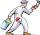If one liter of pants covers an area of 5 m2, how much paint is needed to cover: a) rectangular swimming pool With dimensions 4m x 3m x 2.5m (the Inside walls and the floor only) b) the Inside walls and floor of a cylindrical reservoir with a diameter of
• The roomThe room has a cuboid shape with dimensions: length 50m and width 60dm and height 300cm. Calculate how much this room will cost paint (a floor is not painted) if the window and door area is 15% of the total area and 1m2 cost 15 euro.
• Pool tilesThe pool is 25m long, 10m wide, and 160cm deep. How many m2 of tiles will be needed on the walls and the pool? How many tiles are needed when 1 tile has a square shape with a 20cm side? How much does it cost when 1m2 of tiles costs 258 Kc?
• Traffic cones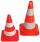Forty identical traffic cones with a base diameter d = 3 dm and a height v = 6 dm should be painted on the outside with orange paint (without base). How many crowns do we pay for color? If we need 50 cm ^ 3 of paint to paint, 1m2 and 1l of paint costs CZ
• Church roof 2The roof has the shape of a rotating cone shell with a base diameter of 6 m and a height of 2.5 m. How many monez (CZK) will cost the roof cover sheet if 1 m2 of metal sheet costs 152 CZK and if you need 15% extra for joints, overlays and waste?
• PaintingThe room is long 50 meters and wide 60 dm and 300 cm high. Calculate how much it will cost to paint if the area of windows and doors is 15% of the total area. One square meter cost 50cents.
• Room dimensions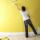Room dimensions are 5m and 3.5m. Room height is 2.85m. Paint the room (even with the ceiling). There will be 2 layers. Doors and windows have a total of 2.5 m2. One box of paint is enough for 6m2. How many boxes of paint are needed? How much do we pay if
• Pavilion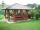The rectangular pavilion with dimensions 3.5 m and 2.75 m to be paved with square tiles of side 25 cm price of CZK 22 per 1 piece or rectangular tiles with sides of 20 cm and 15 cm in the price of CZK 11 per 1 pc. Which solution is cheaper (write its pric
• GlassAt the glass shop we have to cut 8 sheets of glass. Each was shaped a square with sides of 18 cm. We paid 44 CZK. How much is a 1 m2 of glass?

Do you have an exciting math question or word problem that you can't solve? Ask a question or post a math problem, and we can try to solve it.

We will send a solution to your e-mail address. Solved examples are also published here. Please enter the e-mail correctly and check whether you don't have a full mailbox.

Money - math problems. Area - math problems.